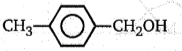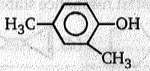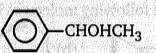NEET Questions Solved

An organic compound having the molecular formula C8H10O on being heated with I2 and dilute NaOH gives a yellow precipitate. The expected compound is:

(a) C6H5CH2CH2OH

(b)(c)(d)(d) $\begin{array}{ccccc}\mathrm{Ph}& -& \stackrel{\mathrm{OH}}{\stackrel{|}{\mathrm{CH}}}& -& {\mathrm{CH}}_{3}\underset{\mathrm{Oxidation}}{\overset{\mathrm{NaOI}}{\to }}\end{array}\begin{array}{ccccc}\mathrm{Ph}& -& \stackrel{\mathrm{O}}{\stackrel{\parallel }{\mathrm{C}}}& -& {\mathrm{CH}}_{3}\end{array}\underset{\mathrm{Haloform}}{\overset{{\mathrm{I}}_{2}+\mathrm{NaoH}}{\to }}$Ph-CO2 + CHI3$↓$

Difficulty Level:

• 12%
• 12%
• 35%
• 43%